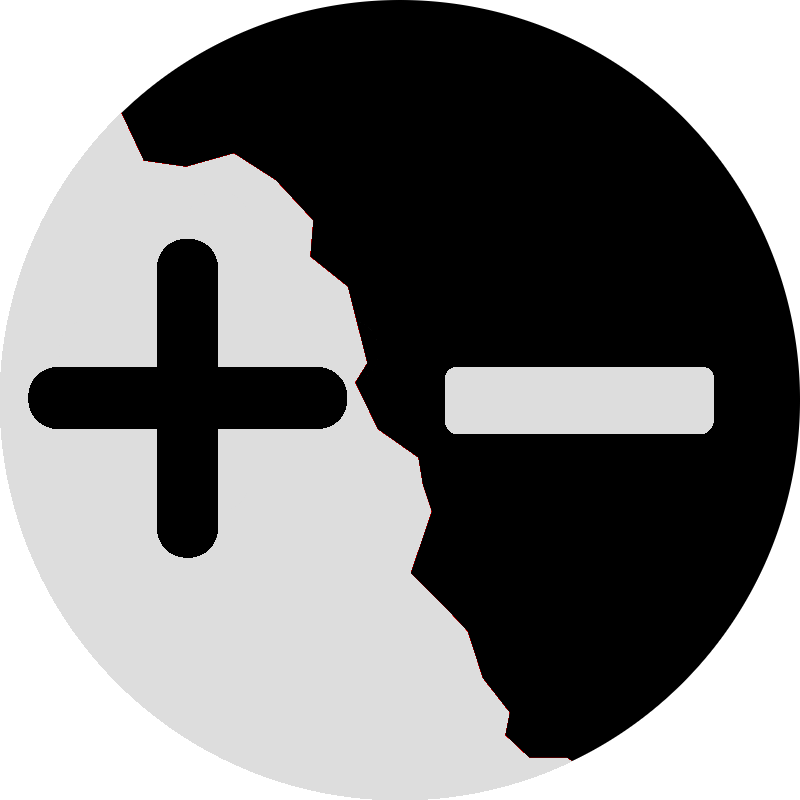# MathPure knowledge.

### Negative Snell's Law Summer 2013

In the Summer of 2013, I took part in ICERM's undergraduate REU. Under the mentorship of Prof. Chaim Goodman Strauss (U. of Arkansas) and Diana Davis (P.h.D, Brown '13), three of us (myself, Kelsey DiPietro, and Jenny Rustad) investigated a dynamical system in planar geometry. For a brief overview of the problem and some of our results, have a look at the talk slides. For more in-depth study, have a look at the preprint of the paper (on arXiv).

### Simplicial Chip Firing Summer 2012

In the Summer of 2012, I worked under the mentorship of Prof. Caroline Klivans (Brown) to investigate an open problem in algebraic topology. For a brief overview of the problem, have a look at the talk slides. It includes screenshots of a Java/Processing application written for the project. For more in-depth study, have a look at the summary paper.

### Algebraic Topology MA241

Homotopy, fundamental group, homology.

### Functions of Several Variables (I) MA113

Point set topology, manifolds, differentiation, measure theory, Lebesgue integration, etc.

### Analysis: Functions of One Variable MA101

Completeness properties of the real number system, topology of the real line, basic theorems in calculus, infinite series, etc.

### Number Theory MA156

Unique factorization, prime numbers, modular arithmetic, quadratic number fields, finite fields, p-adic numbers, etc.

### Topics in Abstract Algebra MA154

Galois theory and representation theory.

### Abstract Algebra MA153

Groups, rings, fields, etc.

### Linear Algebra MA52

Vector spaces, linear transformations, matrices, systems of linear equations, bases, projections, rotations, determinants, and inner products, etc.

### Honors Calculus MA35

Vector analysis, partial differentiation, multiple integration, line integrals, Green's theorem, Stokes' theorem, the divergence theorem, etc.